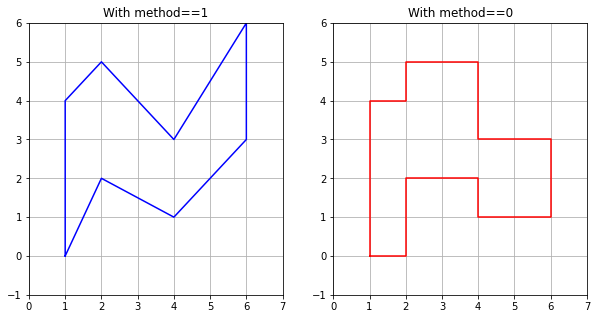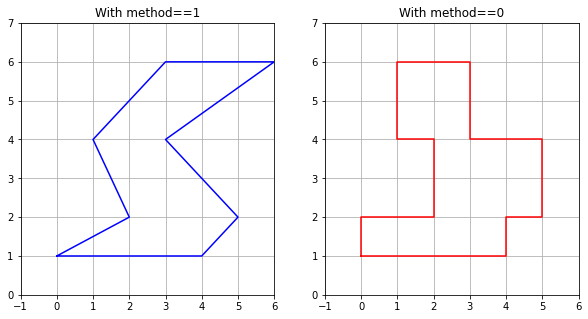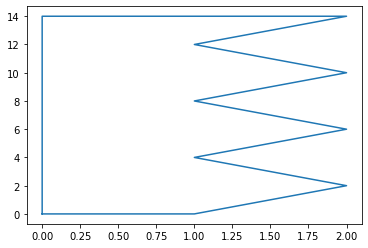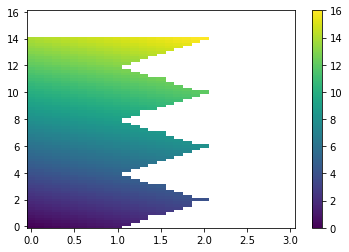# Ruled Rectangles¶

This Jupyter notebook can be found in this collection of Clawpack apps as the file $CLAW/apps/notebooks/amrclaw/RuledRectangles.ipynb. To run this notebook, install Clawpack, and clone the apps repository. A static view of this and other notebooks can be found in the Clawpack Gallery of Jupyter notebooks. See the module clawpack.amrclaw.region_tools for the tools described below. This notebook has also been adapted to the documentation page www.clawpack.org/ruled_rectangles.html. In past versions of AMRClaw and GeoClaw one could specify "refinement regions" as rectangles over which a minlevel and maxlevel are specified (perhaps over some time interval), as described at http://www.clawpack.org/regions.html. Allowing only rectangles made it very easy to check each cell for inclusion in a region, but is a severe restriction -- often a number of rectangles must be used to follow a complicated coastline, for example, or else many points ended up being refined that did not require it (e.g. onshore points that never get wet in a GeoClaw simulation). Starting in Version 5.7.0, we have introduced a new data structure called a "Ruled Rectangle" that is a special type of polygon for which it is also easy to check whether a given point is inside or outside, but that is much more flexible than a rectangle. It is a special case of a "ruled surface", which is a surface in 3D that is bounded by two curves, each parameterized by$s$from$s_1$to$s_2$, and with the surface defined as the union of all the line segments connecting one curve to the other for each value of$s$in$[s_1,s_2]$. A Ruled Rectangle is a special case in which each curve lies in the$x$-$y$plane and either$s=x$for some range of$x$values or$s=y$for some range of$y$values. If$s=x$, for example, then the line segments defining the surface are intervals$y_{\scriptstyle lower}(x) \leq y \leq y_{\scriptstyle upper}(x)$for each$x$over some range$x_1 \leq x \leq x_2$. It is easy to check if a given$(x_c,y_c)$is in this region: it is if$x_1 \leq x_c \leq x_2$and in addition$y_{\scriptstyle lower}(x_c) \leq y_c \leq y_{\scriptstyle upper}(x_c)$. The class region_tools.RuledRectangle supports a subset of ruled rectangles defined by a finite set of$s$values along a coordinate line, e.g.$s < s < s < \cdots < s[N]$and for each$s[k]$two values lower[k] and upper[k]. If rr is an instance of this class then rr.s, rr.lower, and rr.upper contain these arrays. Whether s corresponds to x or y is determined by: • If rr.ixy in [1, 'x'] then s gives a set of$x$values, • If rr.ixy in [2, 'y'] then s gives a set of$y$values. The points specified can then be connected by line segments to define a Ruled Rectangle, and this is done if rr.method == 1 (piecewise linear). On the other hand, if rr.method == 0 (piecewise constant) then the values lower[k], upper[k] are used as the bounds for all s in the interval$s[k] \leq s \leq s[k+1]$(for$k = 0,~1,~\ldots,~N-1$). In this case the values lower[N], upper[N] are not used. This also defines a polygon, but one that consists of a set of stacked boxes. The advantage of the latter form is that it is slightly easier to check if a point is in the Ruled Rectangle since no linear interpolation is required along the edges. Also for some applications we want the Ruled Rectangle to exactly cover a contiguous set of finite volume grid cells, which has the shape of a set of stacked boxes. ## Examples¶ Some simple examples follow: In : %matplotlib inline  In : from pylab import * from matplotlib import cm from IPython.display import Image import os,sys  Import some custom modules: • region_tools includes the RuledRectangle class, • pcolorcells is used to better plot cell-averaged data, see ../visclaw/pcolorcells.ipynb In : from clawpack.amrclaw import region_tools from clawpack.visclaw.plottools import pcolorcells  Define a Ruled Rectangle by specifying a set of points: In : rr = region_tools.RuledRectangle() rr.ixy = 1 # so s refers to x, lower & upper are limits in y rr.s = array([1,2,4,6]) rr.lower = array([0,2,1,3]) rr.upper = array([4,5,3,6])  Setting RR.method to 1 or 0 gives a Ruled Rectangle in which the points specified above are connected by lines or used to define stacked boxes. Both are illustrated in the figure below. Note that we use the method RR.vertices() to return a list of all the vertices of the polygon defined by RR for plotting purposes. In : figure(figsize=(10,5)) subplot(121) rr.method = 1 xv,yv = rr.vertices() plot(xv,yv,'b') grid(True) axis([0,7,-1,6]) title('With method==1') subplot(122) rr.method = 0 xv,yv = rr.vertices() plot(xv,yv,'r') grid(True) axis([0,7,-1,6]) title('With method==0')  Out: Text(0.5, 1.0, 'With method==0')In the plots above the s values correspond to x = 1, 2, 4, 6, and the lower and upper arrays define ranges in y. If we set rr.ixy = 2 or 'y', then the s values will instead correspond to y = 1, 2, 4, 6 and the lower and upper will define ranges in x. This is illustrated in the plots below. In : rr.ixy = 2 # so s refers to y, lower & upper are limits in x figure(figsize=(10,5)) subplot(121) rr.method = 1 xv,yv = rr.vertices() plot(xv,yv,'b') grid(True) axis([-1,6,0,7]) title('With method==1') subplot(122) rr.method = 0 xv,yv = rr.vertices() plot(xv,yv,'r') grid(True) axis([-1,6,0,7]) title('With method==0')  Out: Text(0.5, 1.0, 'With method==0')## Relation to convex polygons¶ Note that the polygons above are not convex, but clearly some Ruled Rectangles would be convex. Conversely, any convex polygon can be expressed as a Ruled Rectangle --- simply order the vertices so that the$x$values are increasing, for example, and use these as the s values in a RuledRectangle with ixy='x'. Then for each$x$there is a connected interval of$y$values that lie within the polygon (by convexity), so this defines the lower and upper values. (Or you could start by ordering vertices by increasing$y$values and similarly define a RuledRectangle with ixy='y'.) So a RuledRectangle is a nice generalization of a convex polygon for which it is easy to check inclusion of an arbitrary point. ## Other attributes and methods¶ ### ds¶ If the points s[k] are equally spaced then ds is the spacing between them. This makes it quicker to determine what two points an arbitrary value of$s\$ lies between when determining whether a large set of points are inside or outside the Ruled Rectangle, rather than having to search.

The Ruled Rectangle defined above has unequally spaced points and the ds attribute is set to -1 in this case.

In :
rr.ds

Out:
-1

### slu¶

Rather than specifying s, lower, and upper separately, you can specify an array slu with three columns in defining a RuledRectangle, and such an array is returned by the slu method:

In :
rr.slu()

Out:
array([[1, 0, 4],
[2, 2, 5],
[4, 1, 3],
[6, 3, 6]])

Here's an example defining a RuledRectangle via slu:

In :
slu = vstack((linspace(0,14,8), zeros(8), [1,2,1,2,1,2,1,2])).T
print('slu = \n', slu)

rr = region_tools.RuledRectangle(slu=slu)
rr.ixy = 2
rr.method = 1
xv,yv = rr.vertices()
plot(xv,yv)

slu =
[[ 0.  0.  1.]
[ 2.  0.  2.]
[ 4.  0.  1.]
[ 6.  0.  2.]
[ 8.  0.  1.]
[10.  0.  2.]
[12.  0.  1.]
[14.  0.  2.]]

Out:
[<matplotlib.lines.Line2D at 0x115da6160>]### bounding_box¶

rr.bounding_box() returns the smallest rectangle [x1,x2,y1,y2] containing RR:

In :
rr.bounding_box()

Out:
[0.0, 2.0, 0.0, 14.0]

### mask_outside¶

If X,Y are 2D numpy arrays defining (x,y) coordinates on a grid, then RR.mask_outside(X,Y) returns a mask array M of the same shape as X,Y that is True at points outside the Ruled Rectangle.

In :
x = linspace(0,3,31)
y = linspace(0,16,81)
X,Y = meshgrid(x,y)
Z = X + Y # sample data values to plot

pcolorcells(X,Y,Zm)
colorbar()

Out:
<matplotlib.colorbar.Colorbar at 0x115ebcc50>RR.write(fname) writes out the slu array and other attributes to file fname, and RR.read(fname) can be used to read in such a file. You can also specify fname when instantiating a new Ruled Rectangle:

In :
rr.write('RRzigzag.data')

In :
rr2 = region_tools.RuledRectangle('RRzigzag.data')
rr2.slu()

Out:
array([[ 0.,  0.,  1.],
[ 2.,  0.,  2.],
[ 4.,  0.,  1.],
[ 6.,  0.,  2.],
[ 8.,  0.,  1.],
[10.,  0.,  2.],
[12.,  0.,  1.],
[14.,  0.,  2.]])

Here's what the file looks like:

In :
lines = open('RRzigzag.data').readlines()
for line in lines:
print(line.strip())

2   ixy
1   method
2    ds
8    nrules
0.000000000   0.000000000   1.000000000
2.000000000   0.000000000   2.000000000
4.000000000   0.000000000   1.000000000
6.000000000   0.000000000   2.000000000
8.000000000   0.000000000   1.000000000
10.000000000   0.000000000   2.000000000
12.000000000   0.000000000   1.000000000
14.000000000   0.000000000   2.000000000


Note that this Ruled Rectangle has equally spaced points and so ds = 2 is the spacing.

### make_kml¶

rr.make_kml() can be used to create a kml file that can be opened on Google Earth to show the polygon defined by rr. This assumes that x corresponds to longitude and y to latitude and is designed for GeoClaw applications. Several optional arguments can be specified: fname, name, color, width, verbose.

### A GeoClaw AMR flag region¶

The figure below shows a Ruled Rectangle designed to cover Admiralty Inlet, the water way between the Kitsap Peninsula and Whidbey Island connecting the Strait of Juan de Fuca to lower Puget Sound. For some tsunami modeling problems it is important to cover this region with a finer grid than is needed elsewhere.

In :
Image('http://www.clawpack.org/gallery/_static/figures/RuledRectangle_AdmiraltyInlet.png', width=400)

Out: